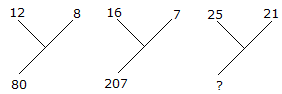# Verbal Reasoning - Character Puzzles - Discussion

### Discussion :: Character Puzzles - Character Puzzles 1 (Q.No.14)

14.

Which one will replace the question mark ?[A]. 184 [B]. 210 [C]. 241 [D]. 425

Explanation:

(12)2 - (8)2 = 80

and (16)2 - (7)2 = 207

Therefore (25)2 - (21)2 = 184.

 M.K.Jain said: (Oct 2, 2012) (12+8) (12-8) =80. (16+7) (16-7) =207. (25+21) (25-21) =184.

 Fayaz said: (Jan 6, 2017) 12 - 8 = 4. 4 * 12 = 48. 4 * 8 = 32. 48 + 32 = 80. 25-21 = 4. 25 * 4 = 100. 21 * 4 = 84. 100 + 84 = 184.

 Siva Prasanth said: (Jun 6, 2017) 12 + 8 = 20 then 20 * 4 = 80. 16 + 7 = 23 then 23 * 9 = 207. 21 + 25 = 46 then 46 * 4 = 184.

 Arif Hassan said: (Jun 13, 2020) Thanks for explaining the solution.

 Zain Bilal said: (Apr 10, 2021) 12+8 = 20*(12-8) = 80. 16+7 = 23*(16-7) = 207. 25+21 = 46 * (25-21) = 184.

 Suresh said: (Sep 15, 2021) 12 * 12 - 8 * 8 = 80, 16 * 16 - 7 * 7 = 207, 25 * 25 - 21 * 21 = 184.

 Suresh said: (Sep 15, 2021) 12 * 12 - 8 * 8 = 80, 16 * 16 - 7 * 7 = 207, 25 * 25 - 21 * 21 = 184.